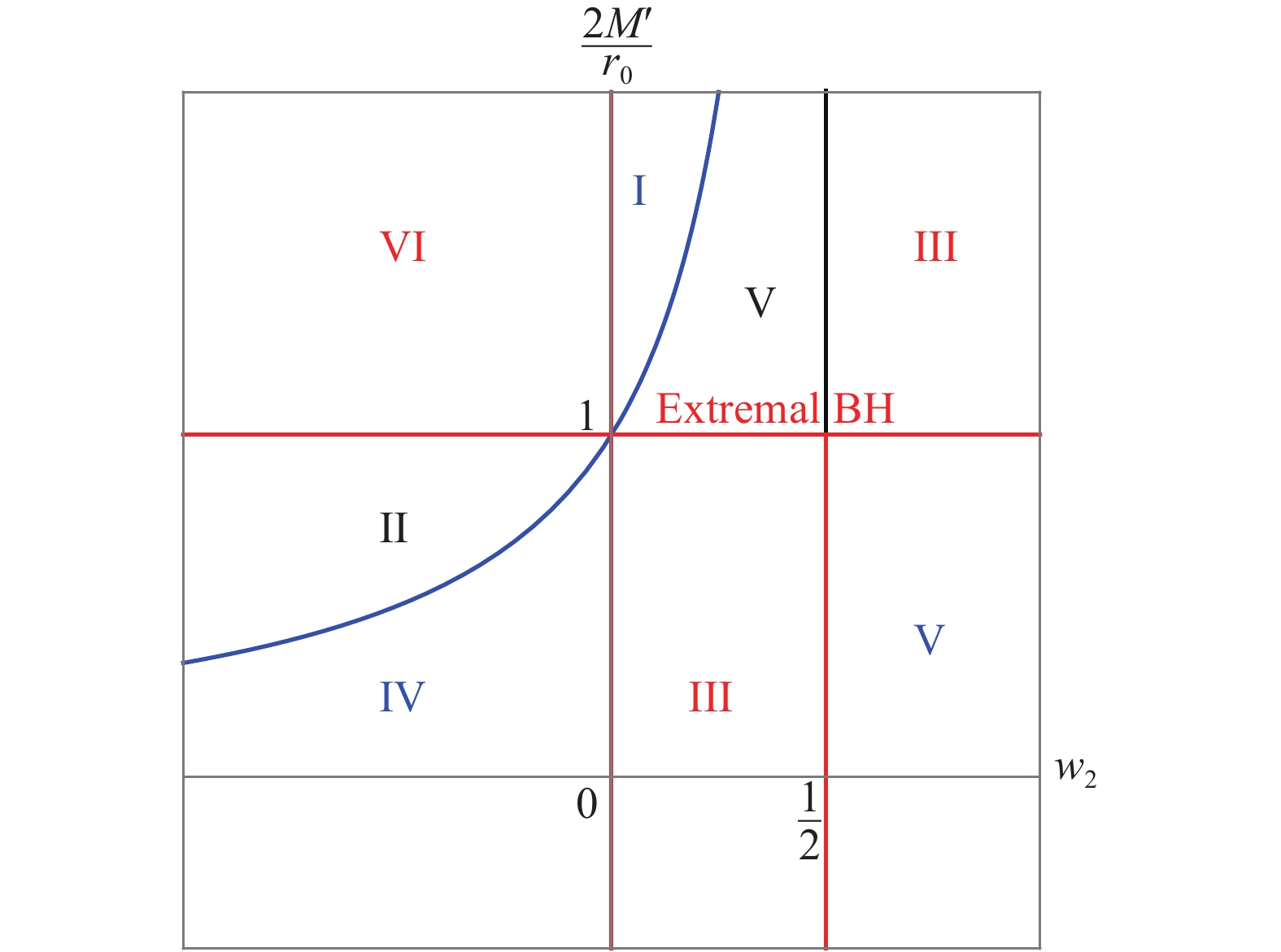# Simple black holes with anisotropic fluid

• We study a spherically symmetric spacetime made of an anisotropic fluid whose radial equation-of-state is given by $p_1 = -\rho$. This case allows analytic solutions and is a good example for studying the static configuration of a black hole plus matter. For a given equation-of-state parameter $w_2 = p_2/\rho$for angular directions, we find the exact solutions of the Einstein equation described by two parameters. We classify the solutions into six types based on the behavior of the metric function. Depending on the parameters, the solutions can have event and cosmological horizons. One of the solution types corresponds to a generalization of the Reissner-Nordström black hole, the thermodynamic properties for which are obtained in a simple form. The solutions are stable under radial perturbations.
••Get Citation
Inyong Cho and Hyeong-Chan Kim. Simple black holes with anisotropic fluid[J]. Chinese Physics C, 2019, 43(2): 1-7. doi: 10.1088/1674-1137/43/2/025101
Inyong Cho and Hyeong-Chan Kim. Simple black holes with anisotropic fluid[J]. Chinese Physics C, 2019, 43(2): 1-7.Milestone
Received: 2018-07-09
Revised: 2018-11-07
Article Metric

Article Views(246)
PDF Downloads(8)
Cited by(0)
Policy on re-use
To reuse of subscription content published by CPC, the users need to request permission from CPC, unless the content was published under an Open Access license which automatically permits that type of reuse.
###### 通讯作者: 陈斌, bchen63@163.com
• 1.

沈阳化工大学材料科学与工程学院 沈阳 110142

Title:
Email:

## Simple black holes with anisotropic fluid

###### Corresponding author: Hyeong-Chan Kim, hckim@ut.ac.kr
• 1. School of Liberal Arts, Seoul National University of Science and Technology, Seoul 01811, Korea
• 2. School of Liberal Arts and Sciences, Korea National University of Transportation, Chungju 27469, Korea

Abstract: We study a spherically symmetric spacetime made of an anisotropic fluid whose radial equation-of-state is given by $p_1 = -\rho$. This case allows analytic solutions and is a good example for studying the static configuration of a black hole plus matter. For a given equation-of-state parameter $w_2 = p_2/\rho$for angular directions, we find the exact solutions of the Einstein equation described by two parameters. We classify the solutions into six types based on the behavior of the metric function. Depending on the parameters, the solutions can have event and cosmological horizons. One of the solution types corresponds to a generalization of the Reissner-Nordström black hole, the thermodynamic properties for which are obtained in a simple form. The solutions are stable under radial perturbations.

### HTML1.   Introduction2.   Field equation3.   Analytic solutions4.   Classification and black hole solutions5.   Stability6.   Summary
PDF查看关注分享

Top

### 目录/DownLoad:  Full-Size Img  PowerPoint